• r语言实现一元线性回归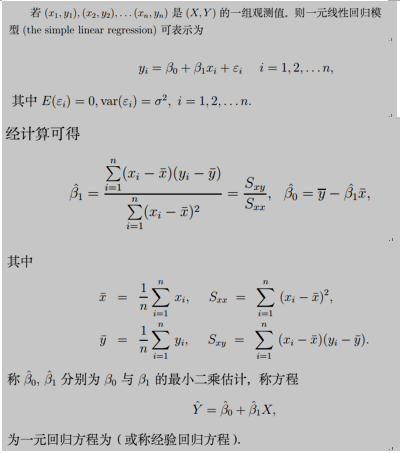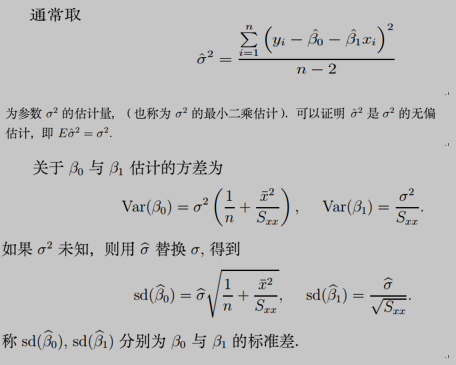常用三种检验方法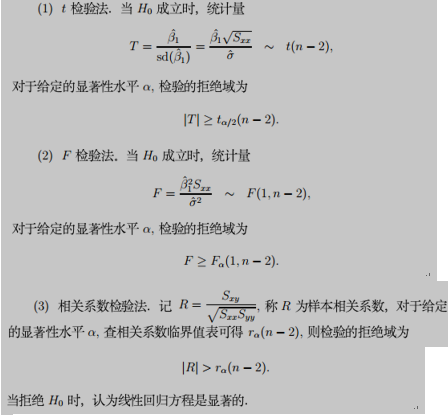X<-matrix(c(
194.5, 20.79, 1.3179, 131.79,
194.3, 20.79, 1.3179, 131.79,
197.9, 22.40, 1.3502, 135.02,
198.4, 22.67, 1.3555, 135.55,
199.4, 23.15, 1.3646, 136.46,
199.9, 23.35, 1.3683, 136.83,
200.9, 23.89, 1.3782, 137.82,
201.1, 23.99, 1.3800, 138.00,
201.4, 24.02, 1.3806, 138.06,
201.3, 24.01, 1.3805, 138.05,
203.6, 25.14, 1.4004, 140.04,
204.6, 26.57, 1.4244, 142.44,
209.5, 28.49, 1.4547, 145.47,
208.6, 27.76, 1.4434, 144.34,
210.7, 29.04, 1.4630, 146.30,
211.9, 29.88, 1.4754, 147.54,
212.2, 30.06, 1.4780, 147.80),
ncol=4, byrow=T,
dimnames = list(1:17, c("F", "h", "log", "log100")))
forbes<-as.data.frame(X)
plot(forbes$F, forbes$log100)
lm.sol<-lm(log100 ~ F, data=forbes)
summary(lm.sol)
Call:
lm(formula = log100 ~ F, data = forbes)

Residuals:
Min       1Q   Median       3Q      Max
-0.32261 -0.14530 -0.06750  0.02111  1.35924

Coefficients:
Estimate Std. Error t value Pr(>|t|)
(Intercept) -42.13087    3.33895  -12.62 2.17e-09 ***
F             0.89546    0.01645   54.45  < 2e-16 ***
---
Signif. codes:  0 ‘***’ 0.001 ‘**’ 0.01 ‘*’ 0.05 ‘.’ 0.1 ‘ ’ 1

Residual standard error: 0.3789 on 15 degrees of freedom
Multiple R-squared:  0.995,	Adjusted R-squared:  0.9946
F-statistic:  2965 on 1 and 15 DF,  p-value: < 2.2e-16

abline(lm.sol)   将得到的直线方程画在散点图上
y.res<-residuals(lm.sol) 计算回归方程的残差
plot( y.res)
new <- data.frame(x = 0.16)   预测x=0.16时Y的值并给出相应的预测区间lm.pred<-predict(lm.sol, new, interval="prediction", level=0.95) lm.pred
fit
lwr upr[1,] 49.42639 46.36621 52.48657

﻿﻿
﻿﻿

展开全文• 使用R语言进行一元回归我们通过一个例子来介绍通过R语言进行一元回归的方法例子：为研究某实验过程中，温度x（℃）对产品得率（%）的影响，测得数据如下： 温度x（℃） 100 110 120 130 140 150 160 170 ...
使用R语言进行一元回归

我们通过一个例子来介绍通过R语言进行一元回归的方法

例子：为研究某实验过程中，温度x（℃）对产品得率（%）的影响，测得数据如下：

温度x（℃）
100
110
120
130
140
150
160
170
180
190
得率Y（%）
45
51
54
61
66
70
74
78
85
89

说明：该例子来自盛骤等老师编写的第四版概率论与数理统计书籍

首先，我们把x,Y数值之间的联系先用散点图描绘出来：

p = c(100,110,120,130,140,150,160,170,180,190)
q = c(45,51,54,61,66,70,74,78,85,89)

plot(p,q,type="p",col="blue",xlab="x",ylab="Y")

运行结果：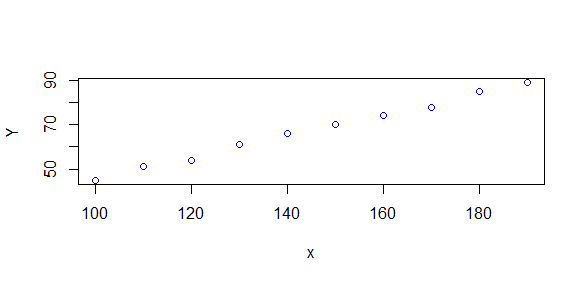从上面散点图我们可以看出，x与Y之间的相关性很强，近似一种线性关系。一元回归的目的就是拟合出一条回归直线，使得散点图上的每一点与这条直线的纵向距离最短。通过拟合出的回归直线，可以用于预测。
设：Y=a+bx+ε，ε N(0,σ2)<!--//--><![CDATA[//><!--
Y=a+bx+ε，ε~N(0,σ^2)
//--><!]]>
对参数a,b进行估计：
其中： b̂ =∑ni=1(xi−x̅)(yi−y̅)∑ni=1(xi−x̅)2<!--//--><![CDATA[//><!--
b ̂ = \dfrac{\sum_{i=1}^n (x_i-x ̅)(y_i-y ̅)}{\sum_{i=1}^n (x_i-x ̅)^2}
//--><!]]>â =y̅−b̂ x̅<!--//--><![CDATA[//><!--
a ̂ =y ̅-b ̂x ̅
//--><!]]>y=â +b̂ x̅<!--//--><![CDATA[//><!--
y=a ̂ + b ̂x ̅
//--><!]]> 即为Y关于x的回归方程。
现在，我们使用R语言来求参数a和b 方法一：

p = c(100,110,120,130,140,150,160,170,180,190)
q = c(45,51,54,61,66,70,74,78,85,89)

plot(p,q,type="p",col="blue",xlab="x",ylab="Y")

lxy<-function(x,y){n=length(x);sum(x*y)-sum(x)*sum(y)/n}  #自定义函数
b = lxy(p,q)/lxy(p,p)
a = mean(q)-b*mean(p)
print(b)
print(a)
lines(p,a+b*p)

运行结果：

 0.4830303
 -2.739394

函数图像：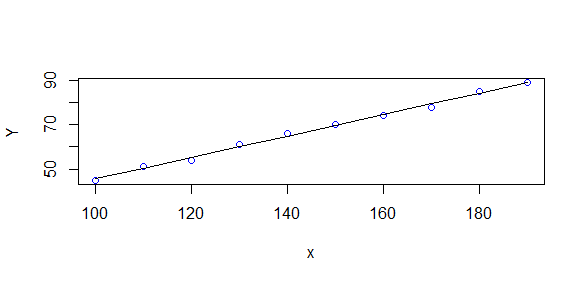即：回归方程为Y=−2.739394+0.4830303∗x<!--//--><![CDATA[//><!--
Y=-2.739394+0.4830303*x
//--><!]]>

方法二：（简单，推荐）

p = c(100,110,120,130,140,150,160,170,180,190)
q = c(45,51,54,61,66,70,74,78,85,89)

r=lm(q~1+p)
print(summary(r))

运行结果：

Call:
lm(formula = q ~ 1 + p)

Residuals:
Min      1Q  Median      3Q     Max
-1.3758 -0.5591  0.1242  0.7470  1.1152

Coefficients:
Estimate Std. Error t value Pr(>|t|)
(Intercept) -2.73939    1.54650  -1.771    0.114
p            0.48303    0.01046  46.169 5.35e-11 ***
---
Signif. codes:
0 ‘***’ 0.001 ‘**’ 0.01 ‘*’ 0.05 ‘.’ 0.1 ‘ ’ 1

Residual standard error: 0.9503 on 8 degrees of freedom
Multiple R-squared:  0.9963,    Adjusted R-squared:  0.9958
F-statistic:  2132 on 1 and 8 DF,  p-value: 5.353e-11


R中提供了lm( )函数来进行回归分析，使用summary( )可以得到回归结果的所有参数。其中，p后面的三个星号***表示参数的估计是很显著的。
展开全文• 一元线性回归分析 首先介绍回归分析中最基础的情况：一元线性回归分析。它规定模型ｆ函数只能是ｙ=k*x+b的形式，即只使用一个变量ｘ（故称为一元）的线性形式来预测目标变量ｙ。 6.1.1引例 利用某网站历次促销...
一元线性回归分析
首先介绍回归分析中最基础的情况：一元线性回归分析。它规定模型ｆ函数只能是ｙ=k*x+b的形式，即只使用一个变量ｘ（故称为一元）的线性形式来预测目标变量ｙ。
6.1.1引例
利用某网站历次促销活动中促销让利费用和销售金额的数据（单位是十万元），将使用该数据集来说明线性回归分析的应用。
使用如下语句来绘制其散点图：
cost<-c(1.8,1.2,0.4,0.5,2.5,2.5,1.5,1.2,1.6,1.0,1.5,0.7,1.0,0.8)
sales<-c(104,68,39,43,127,134,87,77,102,65,101,46,52,33)
data<-data.frame(cost=cost,sales=sales)
plot(data,pch=16,xlab="cost促销让利费用（十万元）",ylab="sales 促销销量（十万元)")
sol.lm<-lm(sales~cost,data)
abline(sol.lm,col="red")

由上图可以看出，促销让利费用cost变量和促销销量sales变量之间呈直线形式。
６.１.２　一元线性回归分析的原理及Ｒ语言实现
１.　最小二乘法
最小二乘法旨在使获得的模型最大限度地拟合样本数据
２．样本方差和协方差
３.　计算模型的参数ｋ和ｂ
（１）估算参数ｋ和ｂ的典型值
(k<-cov(cost,sales)/cov(cost,cost))#模型参数ｋ
(b<-mean(sales)-k*mean(cost))        #模型参数ｂ
也可使用回归方程建立函数lm来计算。代码如下：
(sol.lm<-lm(sales~cost,data))
Call:
lm(formula= sales ~ cost, data = data)

Coefficients:
(Intercept)         cost
13.82        48.60
（２）估算参数ｋ和ｂ的取值范围
在计算得到的回归模型ｙ＝k*x+b中，系数ｂ和ｋ只是一个真实模型的典型估算值，其估算范围是：
[ki-sd(ki)tα/2(n-2),ki+sd(ki)tα/2(n-2)]
其中，k0表示回归模型ｙ＝ｋ×ｘ＋ｂ中的ｂ，k1表示ｋ。sd(ki)是参数的标准差，可以使用summary(sol.lm)$coefficients[,2]直接读取，代码如下： df<-sol.lm$df.residual
alpha=0.05
left<-summary(sol.lm)$coefficients[,1]-summary(sol.lm)$coefficients[,2]*qt(1-alpha/2,df);left
(Intercept)        cost
1.667702  40.182861
right<-summary(sol.lm)$coefficients[,1]+summary(sol.lm)$coefficients[,2]*qt(1-alpha/2,df);right
(Intercept)        cost
25.97978   57.01138
在上述代码中，df为自由度，等于样本数n减去自变量数p,再减１，即为n-2，该自由度可以通过sol.lm$df.residual直接读取，alpha是置信度，典型取值0.05,left是取值范围的最小值，right是取值范围的最大值。 ４. 衡量相关程度 （１）相关系数ｒ和判定系数r2 对于引例，可以使用如下代码来计算相关参数。 (r<-cor(cost,sales)) #相关系数r (r2<-r^2) #判定系数r2 在ｌｍ函产生的结果中也包含了判定系数ｒ的信息。 summary(sol.lm) Call: lm(formula= sales ~ cost, data = data) Residuals: Min 1Q Median 3Q Max -19.701 -3.566 1.430 4.873 14.281 Coefficients: Estimate Std. Error t valuePr(>|t|) (Intercept) 13.824 5.579 2.478 0.0291 * cost 48.597 3.862 12.584 2.84e-08 *** --- Signif.codes: 0 ‘***’ 0.001 ‘**’ 0.01 ‘*’ 0.05‘.’ 0.1 ‘ ’ 1 Residualstandard error: 9.106 on 12 degrees of freedom MultipleR-squared: 0.9296, Adjusted R-squared: 0.9237 F-statistic:158.4 on 1 and 12 DF, p-value: 2.843e-08 上述的Multiple R-squared就是判定系数ｒ２，该系数也可以使用summary(sol.lm)$r.squared来直接读取。
summary(sol.lm)
Call:
lm(formula= sales ~ cost, data = data)

Residuals:
Min     1Q  Median      3Q    Max
-19.701  -3.566  1.430   4.873  14.281

Coefficients:
Estimate Std. Error t valuePr(>|t|)
(Intercept)  13.824      5.579  2.478   0.0291 *
cost          48.597      3.862 12.584 2.84e-08 ***
---
Signif.codes:  0 ‘***’ 0.001 ‘**’ 0.01 ‘*’ 0.05‘.’ 0.1 ‘ ’ 1

Residualstandard error: 9.106 on 12 degrees of freedom
F-statistic:158.4 on 1 and 12 DF,  p-value: 2.843e-08
上述的Adjusted R-squared就是修正判定系数，该系数也可以使用summary(sol.lm)$adj.r.squared来直接读取。 ５. 回归系数的显著性检验 （１）Ｔ检验 Ｔ检验可以检验各个模型参数是否等于０，并计算其等于０时的概率。一般来说，当p.value小于０.０５，时，可以认定ｋ不会等于０，其模型结果可用并通过了检验。 summary(sol.lm) Call: lm(formula= sales ~ cost, data = data) Residuals: Min 1Q Median 3Q Max -19.701 -3.566 1.430 4.873 14.281 Coefficients: Estimate Std. Error t valuePr(>|t|) (Intercept) 13.824 5.579 2.478 0.0291 * cost 48.597 3.862 12.584 2.84e-08 *** --- Signif.codes: 0 ‘***’ 0.001 ‘**’ 0.01 ‘*’ 0.05‘.’ 0.1 ‘ ’ 1 Residualstandard error: 9.106 on 12 degrees of freedom MultipleR-squared: 0.9296, Adjusted R-squared: 0.9237 F-statistic:158.4 on 1 and 12 DF, p-value: 2.843e-08 也可以使用如下代码直接读取结果: summary(sol.lm)$coefficients[,4]
(Intercept)         cost
2.907896e-022.843305e-08
(2)　Ｆ检验
与Ｔ检验不同，Ｆ检验用于在整体上检验模型参数是否为０，并计算等于０的概率。
summary(sol.lm)
Call:
lm(formula= sales ~ cost, data = data)

Residuals:
Min     1Q  Median      3Q    Max
-19.701  -3.566  1.430   4.873  14.281

Coefficients:
Estimate Std. Error t valuePr(>|t|)
(Intercept)  13.824      5.579  2.478   0.0291 *
cost          48.597      3.862 12.584 2.84e-08 ***
---
Signif.codes:  0 ‘***’ 0.001 ‘**’ 0.01 ‘*’ 0.05‘.’ 0.1 ‘ ’ 1

Residualstandard error: 9.106 on 12 degrees of freedom
F-statistic:158.4 on 1 and 12 DF,  p-value: 2.843e-08
其值为２．８４３ｅ－０８，远小于０．０５，表示通过了Ｆ检验。
在summary(sol.lm)中只给出了样本自由度＼自变量自由度以及Ｆ值，可以使用如下代码来直接读取Ｆ检验的ｐ-value值。
(f<-summary(sol.lm)$fstatistic) (df1<-summary(sol.lm)$fstatistic)
(df2<-summary(sol.lm)$fstatistic) pf(f,df1,df2,lower.tail=F) value 2.843305e-08 或者 1-pf(f,df1,df2) value 2.843305e-08 ６. 模型误差（残差） 回归模型sol.lm的误差（残差residuals）,可用于体现样本点模型预测值与实际数据的差异程度，可通过sol.lm$residuals直接读取误差数据。对已一个正确的回归模型，其误差要服从正态分布。
shapiro.test(sol.lm$residuals) Shapiro-Wilknormality test data: sol.lm$residuals
W= 0.9669, p-value = 0.8329
说明残差服从正态分布。
残差的标准误（Residual standard error）可以从整体上体现一个模型的误差情况，它可以用于不同模型间性能的对比。
summary(sol.lm)
Call:
lm(formula= sales ~ cost, data = data)

Residuals:
Min     1Q  Median      3Q    Max
-19.701  -3.566  1.430   4.873  14.281

Coefficients:
Estimate Std. Error t valuePr(>|t|)
(Intercept)  13.824      5.579  2.478   0.0291 *
cost          48.597      3.862 12.584 2.84e-08 ***
---
Signif.codes:  0 ‘***’ 0.001 ‘**’ 0.01 ‘*’ 0.05‘.’ 0.1 ‘ ’ 1

Residual standarderror: 9.106 on 12 degrees of freedom
F-statistic:158.4 on 1 and 12 DF,  p-value: 2.843e-08
也可以使用summary(sol.lm)\$sigma直接读取。
７.　预测
在Ｒ语言中，使用predict函数可以方便地计算预测值和取值范围。
下面的代码返回原有１４个样本的预测值数据。
predict(sol.lm)
1        2         3         4         5        6         7         8         9        10        11        12        13

101.29856  72.14029 33.26259  38.12230 135.31655135.31655  86.71942  72.14029 91.57914  62.42086  86.71942 47.84173  62.42086

14
52.70144


展开全文数据
• UA MATH571A R语言回归分析实践 一元回归1 NBA球员的工资基础回归分析 571A另一个系列的文章介绍了回归分析的理论，这个系列的文章介绍R语言做回归分析的实践，但不会涉及R语言编程，只是介绍回归分析需要的命令、...
UA MATH571A R语言回归分析实践 一元回归1 NBA球员的工资基础回归分析
571A另一个系列的文章介绍了回归分析的理论，这个系列的文章介绍R语言做回归分析的实践，但不会涉及R语言编程，只是介绍回归分析需要的命令、怎么输入以及怎么解释输出。
NBA球员的draft number与他们的工资之间是有一定的关系的。NBA Draft简单理解就是球队pick心仪的新球员，players selected number 1 overall相当于就是C位，比如2002年的C位就是姚明，他的draft number就是1。所以我们的一个直觉就是新球员的draft number越小（名次越高），他的工资就应该越高。在这个系列的博文中，我们用2017-2018 NBA draft的数据为例，来验证一下我们的直觉，这个数据我上传了的，需要的话可以找来下载。
基础回归分析
首先读取数据，先简单看一下数据在excel里面的样子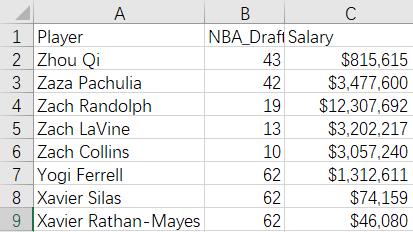setwd("D:/Stat PhD/taking course/summer1/ref/regression")
Data <- read.csv("Salary1.csv", header = TRUE, sep = ",", quote = "\"",
dec = ".", fill = TRUE, comment.char = "")
X <- as.numeric(Data[,2])
Y <- as.numeric(Data[,3])

接下来用lm命令做线性回归就可以了，lm是R语言估计线性模型的函数。第一条命令做工资关于名次的回归，把结果存在ureg01.lm中，第二行输出结果的一些总结信息。
> ureg01.lm <- lm(Y~X)
> summary(ureg01.lm)

Call:
lm(formula = Y ~ X)

Residuals:
Min       1Q   Median       3Q      Max
-9901919 -4761884 -1586787  2215989 27551106

Coefficients:
Estimate Std. Error t value Pr(>|t|)
(Intercept) 10285438     440112   23.37   <2e-16 ***
X            -139730      11404  -12.25   <2e-16 ***
---
Signif. codes:  0 ‘***’ 0.001 ‘**’ 0.01 ‘*’ 0.05 ‘.’ 0.1 ‘ ’ 1

Residual standard error: 6365000 on 649 degrees of freedom
Multiple R-squared:  0.1879,	Adjusted R-squared:  0.1866
F-statistic: 150.1 on 1 and 649 DF,  p-value: < 2.2e-16

第二行以下的内容就是回归结果的总结。call后面这个是回归命令，公式是Y~X，意思就是我们执行了Y关于X的回归。residual后面的是残差的描述性统计，从左到右分别是残差的最小值、25%分位点，中位数，75%分位点以及最大值。Coefficients后面是系数的估计，一元回归只有截距项和解释变量X的系数。第一列是系数的估计值，10285438在这个问题下面的解释是如果有第0名存在，那么工资估计就是这么多，-139730表示名次每降一名，工资平均会降139730，也就是说我们之前的直觉是对的，名次越低工资越低。第二列是系数的估计量的方差，第三列是系数估计量的t统计量，第四列是系数估计量的t检验的p值，这里的t检验原假设是系数为0，因为这两个p值都非常小，所以我们可以很自信地拒绝原假设，认同名次越低工资也会越低的假设。倒数第三行是残差的标准误（标准差）以及相应的自由度，这里一共有651个样本，所以总自由度是650，回归模型占一个自由度，所以误差自由度是649。倒数第二行是R方的结果，第一个R方是多元回归的R方，就是根据残差平方和、回归平方和以及自由度调整计算出来的，一般看这个R方就可以了，这个0.1879表示这个一元线性回归模型可以解释18.79%的工资的变化（也就是说这个模型解释力其实很低，名次对于球员工资没有主要的解释力）；第二个R方是考虑到只要我们不断增加解释变量，第一个R方根据定义的话它就会不断变大，但这种变大没有意义，因为模型可能是过拟合的状态，所以第二个R方相对于第一个会把模型的复杂度考虑进去，在第一个R方的基础上，模型越复杂，第二个R方就会越小。最后一行是对模型整体的检验，它的原假设是截距与X的系数都为0，这里F统计量是150.1，自由度是1和649，p值非常小，说明我们可以拒绝原假设，认为并非截距和X的系数都是0，也就是说这个模型还是有意义的。
现在我们有了第一个解释球员工资的模型，它可以用回归方程表示出来：
$\hat{Y} = 10285438-139730X$
用这个模型我们可以做一些简单的拟合与预测。如果某位球员名次是43名，根据这个模型我们可以估计他的工资应该是
> predict(ureg01.lm,newdata = data.frame(X=43),interval = "conf",level = 0.95)
fit     lwr     upr
1 4277046 3726681 4827410

用来做拟合和预测的都是predict函数，输入第一项是模型对象，我们这个问题的模型对象就是之前估计得到的ureg01.lm，输入第二项newdata = 后面要接的类型数据框，需要用data.frame做一个转换，第三项是选择区间估计的类型，在做predict的时候，区间估计类型有两种，拟合和预测，拟合用conf表示，预测用predict表示，同样的置信水平下拟合的置信区间更短，因为预测会把新样本也看成是随机变量，会让Y的估计值方差更大。第四项是置信水平。输出第一列是fit，也就是拟合值，如果某球员名次是43，那么根据模型估计的工资就是4277046，置信区间是[3726681,4827410]，也就是说我们有95%的把握他的工资会在这个区间内。
再多提一下这个数据框，因为不用数据框或者用的数据框和模型对象的不一致就会报错，如果是多个待拟合对象，我们也要用data.frame把它变成数据框，
> predict(ureg01.lm,newdata = data.frame(X=c(43,44)),interval = "conf",level = 0.95)
fit     lwr     upr
1 4277046 3726681 4827410
2 4137316 3576386 4698245

现在讨论预测，假设有一个球员名次是43名，想要预测他的工资大概是多少，我们也用predict，只是区间估计命令换成pred
> predict(ureg01.lm,newdata = data.frame(X=43),interval = "pred",level = 0.95)
fit      lwr      upr
1 4277046 -8232809 16786901

可以发现第一列的结果和拟合是一样的，只是区间估计的结果不一样了，相同的置信水平下，预测的置信区间会比拟合的更大，但如果这名球员是没有被估计模型的样本包括在内的，一般我们还是需要用预测的。


展开全文统计学 大数据 机器学习
• UA MATH571A R语言回归分析实践 一元回归4 NBA球员的工资Box-Cox变换Full Model模型再诊断总结 上一讲对一元线性回归模型进行了诊断，发现模型主要存在三个问题： 工资关于名次存在负相关，但可能不是线性关系； ...统计学 机器学习
• UA MATH571A R语言回归分析实践 一元回归2 NBA球员的工资方差分析相关性分析 上一讲完成了解释NBA球员工资的一个简单的一元线性回归模型的估计、分析，展示了一下简单的预测，这一讲我们的问题是一元线性回归模型够...统计学 大数据 机器学习
• UA MATH571A R语言回归分析实践 一元回归3 NBA球员的工资残差分析正态性、同方差性的检验欠拟合检验 前两讲已经完成了大致的分析了，我们已经明确了NBA球员名次与工资的负相关关系，接下来我们对一元线性回归模型做...统计学 数据分析 机器学习
• #利用residuals()函数计算回归方程的残差 y.res(lm.sol); plot(y.res) text(12,y.res, labels="残差异常点",adj=1.1) #剔除残差异常点 i; forbes12(X[i!=12, ]) lm12(log100~F, data=forbes12) summary(lm12) ...
• shuju shuju #建立数据 attach(shuju) plot(x,y,main="签发的新保单数目对每周加班时间...summary(shuju.reg) #(3)最小二乘估计(4)求回归标准误差(6)x与y的决定系数(8)回归系数的显著性检验 abline(shuju.reg,统计分析 数据分析 代码
• 本节主要介绍了一元线性回归、多元线性、多项式回归。 重点介绍了summary里面每个参数的意义； 创建训练集、测试集； 多项式poly()函数以及I()函数的使用。 ###########一元线性回归############# > library...r
• R语言一元线性回归 1、准备数据，数据格式为CSV， 2、线性回归脚本， 3、获取回归参数 获取R2 获取截距和斜率 构造回归函数 将公式、R2贴到图形上 输出图形 ...
• R语言解读一元线性回归模型 R的极客理想系列文章，涵盖了R的思想，使用，工具，创新等的一系列要点，以我个人的学习和体验去诠释R的强大。 R语言作为统计学一门语言，一直在小众领域闪耀着光芒。直到大数据的...
• 只有两个变量，做相关性分析，先来个一元线性回归吧 因为未处理的x,y相关性不显著，于是用了ln(1+x)函数做了个处理（发现大家喜欢用ln,log,lg,指数函数做处理），处理完以后貌似就显著了。。虽然R方也比较小 ...
• 高尔顿被誉为现代回归的创始人，"回归效应"的发现源于高尔顿的豌豆遗传试验。在这个试验中，高尔顿发现，并非尺寸大的豌豆，其后代尺寸也大，尺寸小的豌豆，其后代尺寸也小。而是具有一种不同的趋势，...
• 回归分析是一种应用广泛的数理统计方法，它是研究变量与变量之间的相关关系，这种关系大致分为两类：确定性关系（能用函数精确描述）和非确定性关系（不能用函数描述）。 变量间的非确定性关系称为相关关系。 在回归...
• R语言简单（一元）线性回归分析简单举一个例子某商业银行2002年主要业务数据 分行编号 不良贷款（亿元） 各项贷款余额（亿元） 1 0.9 67.3 2 1.1 111.3 3 4.8 173.0 4 3.2 80.8 5 7.8 199.7 6 ......